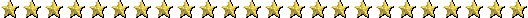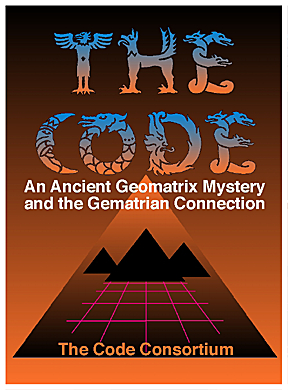"THE CODE" OF CARL MUNCK, AND ANCIENT GEMATRIAN NUMBERS - PART SEVEN

The Crop Circle Connection
by Joseph E. Mason

Background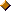I started to follow the crop circle story very closely in 1990.  I have a number of books on the subject and many journals and newsletters.  The two major journals are The Cerealogist and The Circular. I began to see number connections early-on.  I read Joseph Campbell's The Inner Reaches of Outer Space in late 1990.  In a part of the book, he wrote about ancient numbers, such as 108 and 432.  He pointed out how the numbers always add to nine or a multiple of nine.  Similar numbers, I found, were often reported as dimensions of various parts of crop circle formations.  The number nine was quite apparent.

After learning about Carl Munck's "Code" and the Gematrian numbers in 1994, I became more aware of similar numbers within various crop circle formations.  I made contact with Charles Johnson 1996. He is a scholar of ancient numbering systems and has also studied Carl Munck's work.  I mentioned the possible crop circle connection to the ancient numbers to Charles, and later sent him a large packet of information about it.  We planned to do a joint article about the subject. Unfortunately, other plans took priority, so the project was not completed.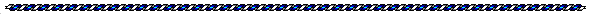Prehistoric Mathematics and British Crop Circles

On August 1, 1997, I received The Cerealogist #19. On pages 9-13, was an article titled, Prehistoric Mathematics & British Crop Circles. The article was originally published in FGK Report, written by Max Seurig and H. Werner Baumann.  It was translated for The Cerealogist by Ute Sayer. I will recount the article here.

The two German researchers analyzed prehistoric stone circles and compared the results to other objects in different parts of the world.  The objects examined were from 600 BC to 2300 BC. The measurements were in meters.  They found a unique order of numbers, which seemed to follow a plan. Equal or related series of numbers were found as azimuth and distance in meters of stone pathways, circumferences of pyramids, the sum of symbols in reliefs, construction features of buildings, etc.  From this, Seurig and Baumann developed "Prehistoric Mathematics." The system shows an unusually high mental-theoretical level, the origin of which is unknown.

Prehistoric Mathematics contains the four main calculations for the division of 360-degree circles and dissected parts of circles defined by their angles, and for triangles and squares, including their angles.  The Pi constant (3.14) is also used.  A "cosmic-related world of numbers" developed, which included the Zodiac and planets known in prehistory.  Most of the calculations lead to the huge time period of the Platonic Year.

The Numbers of the Platonic Year

Since ancient times, the natural constant of the movements of the point of sunrise, the intersection between equator and eclipse at equinox and solstice in one year was 50 ".  In the equation:

1° = 60 '
60 ' = 3600 "

3600 / 50 = 72 (a)

a = 1 earth year
a = 50 "

72 earth years amount to one degree of the Zodiac.  One Platonic day lasts 72 earth years.  One Platonic month covers a period of 30 Platonic days, or 2160 earth years.  Twelve Platonic months give the Platonic year, which lasts 360 x 72 = 25920 earth years.  This system, already used in Chaldaeic astronomy, orientates itself by the numbers 6 and 12, out of which 72 is the product.  Each Platonic month was related to one starsign, or Zodiac sign.  The length of each of the twelve starsigns are different - they are not evenly spaced at 30-degrees each around the 360-degrees of the ecliptic path in the sky.  Therefore, the real duration of time in each starsign is not usually equal to a Platonic month.  Guenther Wachsmuth listed the duration times in a table:

 NUMBER SIGN DEGREES EARTH YEARS TIME PERIOD 1 Virgo 45 3240 13800 to 10500 BC 2 Leo 36 2592 10500 to 7900 BC 3 Cancer 20 1440 7900 to 6500 BC 4 Gemini 28 2016 6500 to 4500 BC 5 Taurus 36 2592 4500 to 1900 BC 6 Aries 25 1800 1900 to 100 BC 7 Pisces 36 2592 100 BC to 2500 AD 8 Aquarius 26 1872 2500 to 4400 AD 9 Capricorn 27 1944 4400 to 6300 AD 10 Sagittarius 32 2304 6300 to 8600 AD 11 Scorpio 30 2160 8600 to 10800 AD 12 Libra 19 1368 10800 to 12100 AD

Only the duration of Scorpio is identical with the length of the Platonic month.  Seurig and Baumann came across such numbers again and again in their research into the constructions of the ancient builders.

The World of Numbers of the Planets

Prehistoric constructors obviously had a knowledge of the planets that had been passed down to them from even more ancient times (see Otto Apelt's Translation of Plato's Timaeus, Leipzig 1919). With their help, the three-dimensional relationships were explained, while the Zodiac served to show the course of time.  The basis was the supposed agreement of the musical seven-step scale and the "seven" of the then-known planets, including the sun and moon.  From this interval, the equation 2 x 3 x 8 x 8 = 384 was determined.  Surprisingly, the distance between moon and Earth is 384,000 kilometers; the 384 in a relationship of 1 : 1000.  By using the Tetractys, the holy four-count of the Pythagorans, the multiple of it is seen in this pyramid:

1
2        3
4              9
8                    27

The multiple of the ratio of the moon could then be used for the other planets.  Apelt's work reveals this table:

 1 Moon 384 x 1 = 384 2 Sun 384 x 2 = 768 3 Venus 384 x 3 = 1152 4 Mercury 384 x 4 = 1536 5 Mars 384 x 8 = 3072 6 Jupiter 384 x 9 = 3456 sum 384 x 27 = 10368 7 Saturn 384 x 27 = 10368 total 384 x 54 = 20736

There are "problems" with figures in this table, such as the figures given for Venus, Mercury, and the sun, but Seurig and Baumann say it is not relevant to their investigations.  The numbers were used as ratios in prehistoric astronomy, similar to the numbers of the Zodiac.

The Crop Circle Connections

To their surprise, Seurig and Baumann found similar relationships of numbers in their study of British crop circles.  They gave a few examples in the article.

Crop Formation of EtchilhamptonThis crop circle formation appeared at Etchilhampton Hill, near Devizes, Wiltshire, in 1993.  Six circles of almost the same size were arranged around a central seventh circle.  Pathways, like spokes on a wheel, connected each of the outer circles to the central circle.  The six spokes divided the formation into sectors of 60-degrees each.  Thus, three axes are built, each connecting its outer two circles with the central circle.  One of the axes ran exactly north-south.

The diameters of the circles varied slightly in size, as did the length of the spokes.  Holding to a reasonable deviation of 0.33 to 0.25%, Seurig and Baumann took the following steps:

First step:  List the diameters of the seven circles, starting at the North circle, and continuing clockwise -

12.00 meters (number of calendar and of religious importance)
11.50 meters
11.40 meters
11.30 meters
11.30 meters
11.30 meters
11.30 meters
_______________
80.10 meters total

Second step:  Calculate the circumference of all circles -

(Pi) 3.14159 x 80.10 overall circumference in meters = 251,6413590
round up to 252.00 meters.

Third step:  Calculate the sum of the spokes, starting from the North, and continuing clockwise -

10.60 meters
9.80 meters
10.00 meters
9.40 meters
9.60 meters
10.40 meters
59.80 m

Round up to 60.00 meters

Forth Step: Multiplication of sum of circumference and spokes -

252.00 meters x 60.00 meters = 15120.00 meters

The first number of astronomical relevance is thus suggested, because 15120 years divide into seven Platonic months:

15120 / 7 = 2160

Fifth step:  Division of 2160 by the number of outer circles -

2160 / 6 = 360 (360-degrees of the Zodiac).

Sixth step:  Diameter of the overall formation -

Axis:         N/S        NE/SW     NW/SE
circle      12.00m      11.00m     11.30m
spoke     10.60m        9.80m     10.00m
circle      11.30m      11.30m     11.30m
spoke       9.40m        9.60m     10.40m
circle      11.30m      11.50m     11.30m
54.60m      53.20m     54.30m

Average:  54.60m + 53.20m + 54.30m = 162.10m

162.10m / 3 = 54.03m

Result rounded to 54m

Seventh step: Overall diameter x sum of spokes -

54.00m x 60.0m = 3240.00m

This figure matches the number of years given in the above chart for the Zodiac sign of Virgo (45-degrees x 72 years = 3240 years).

Eighth step:  Sum of the three axes -

54.60m (N/S) +
53.20m (NE/SW) +
54.30m (NW/SE) +
= 162.10m

Round down to 162m

Ninth step:  Sum of diameters of the circles and of six spokes -

80.00m + 60.00m = 140.00m

Tenth step:  Sum of axes x sum 140.00m

162.00m x 140.00 = 22,680.00m

This figure equals the Platonic year of 25920 earth years minus the years of Virgo, 3240 earth years -

25920 - 3240 = 22680 earth years

The Sequence of Numbers is Relevant

Seurig and Baumann found that in the prehistoric system, decimal places and measure dimensions are not relevant. The sequence of numbers, however, is important.  For example, the diameter of a circle of 12 meters and the length of a spoke of 9.60 meters, adds to 21.60 meters.  It contains the length of a platonic month of 2160 earth years.  The circle diameters of 12.00m + 11.00m + 11.30 = 343, indicate an important number in historical development.  It is seven cubed, 7 x 7 x 7.

It is the opinion of these researchers that a connection exists between measurements of crop circle formations and ancient mathematical-astronomical facts. It becomes more clear when one includes degrees into the calculations.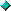1.  Six circles of 360-degrees each are added together:

360 x 6 = 2160

The result shows the known Platonic month or a period of 2160 earth years.2.  Adding the seventh circle:

2160 + 360 = 2520

The result shows the same sequence of numbers as calculated in meters, but ten times larger.3.  Taking the six spokes:

2520 x 6 = 15120

This is again a period of seven time periods of 2160 years each, produced by the number 6 here, whereas the number 60 was included from the measurements to produce the same result in the prior calculation.

Whoever created this crop formation presented a time period of seven time cycles in three ways:

1.  By creating a picture of seven circles.
2.  By calculating on the basis of meters.
3.  By calculating the time on the basis of degrees.

Analysis of this kind can be used to determine the validity of the prehistoric number system and whether it is indeed being incorporated within the crop circle formations.  If it is valid, then it also points toward a view of human development outlined in theosophical and anthroposophical literature.

The "Comet's Tail" Crop Formation

Seurig and Baumann also analyzed the so-called "Comet's Tail" crop formation found in the Wiltshire area in 1994.  The formation revealed a similar systematic structure of number, but in a very different methodical variation.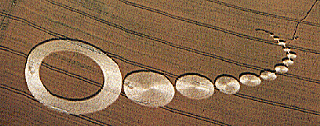Description of the Formation

A "well spring" with an opening, like a mouthpiece or cornucopia, launches, in an elegant curve, twelve circles and one ring.

The ring, furthest way from the mouthpiece, has the largest diameter.  The diameters of the next twelve circles gradually decrease, like "soap bubbles."  The line of twelve circles is divided into sets of five and seven by slightly bigger gaps between the circles.  The calculations start with the smallest circle.

Measurements

Length of the "spring":  7m
Diameters of the group of 7 circles       Length of the gaps between

1.      2.80m                                              1.00m
2.      3.60m                                              0.60m
3.      4.50m                                              0.80m
4.      4.20m                                              0.80m
5.      5.00m                                              0.80m
6.      5.70m                                              0.80m
7       6.60m
32.40m                                              4.80m

Diameters of the group of 5 circles       Length of the gaps between

8.      8.20m                                              0.80m
9.    10.30m                                              0.80m
10.  14.20m                                              0.50m
11.  18.80m                                              0.40m
12.  25.50m                                              0.90m
77.00m                                              3.40m

Plus the width of the ring (13th circle) x 2

18.50m
95.50m (sum of the 5 circles plus the ring)

Length of gap between group of 5 and group of 7 circles

1.90m

Diameter of outer ring 51.80m
Diameter of inner ring -33.30m
= 18.50m

Basis of Interpretation

The researchers viewed the circles coming out of the "spring" as "time-rings" of a calendar of 12 months. The different ring is not included in the first analysis.  The gaps between circles divides the 12 circles into groups of 7 and 5.  Such divisions are also found in mythological literature, with a positive and negative valuation:  7 good or bright periods of time are opposite 5 bad or dark ones, caused by the astrological associations.

Interpretation Method

The various measurements or selected combinations are used as part of the calculations, along with the Pi constant.  The results lead to the Old Astronomy, in which certain numbers adopt a pilot function.  In the case of this crop formation, the gap measurements produced significant additional elements.

Calculations1.  The basic quantity is the "7" (length of the "spring": 7.00m), which plays an important role in astronomy, astrology and cosmology, as well as in mythology.  Taking the set of seven circles, the sum of the diameters is 32.40 meters, or 3240 centimeters.  This signals the starsign of Virgo, the first Platonic month, the new year or birthmonth of the Platonic year.2.  The calculation 32:40m x 7.00m results in 226.8m, the sum of the other eleven Platonic months. 32.40 + 226.80 = 259.20 or 3240 + 22680 = 25920, the whole Platonic year.  Remember too, the same numbers were found within the Etchilhampton crop circle formation.3.  The next calculation is from the sum of the gaps between the circles of the group of 7 circles, 4.80m:

32.40 x 4.80m = 155.52m

This results in more numbers of the Platonic year. 12960 contains six Platonic months of 2160 calendar years each, presumably the first half.  15552 - 12960 = 2592, which stands for a Platonic month developing out of 36-degrees x 72, of which there are three:  Leo, Taurus and Pisces.  This seems to indicate the seventh month of the Platonic year, the starsign Pisces.  Currently, the spring equinox is situated in about the third quarter of Pisces.  Perhaps the number 15552 indicates the present Zodiac Age.

Another solution is possible, and perhaps it is even more valid.  As mentioned above, seven Platonic months were grouped as a time cycle in ancient times.  Such a period ended dramatically in the starsign of Leo about 10,000 to 8,000 BC.  Six time-cycles or Ages totaling 12960 years would contain the Platonic months of Aquarius, Capricorn, Sagittarius, Scorpio, Libra and Virgo. Therefore, the intermediate result in the calculations above, 2592, may indicate the duration in years of the Age of Leo.

The number 2592 is confirmed by another calculation - the differences between the various diameters of the circles:

3.60m - 2.80m = 0.80m
4.50m - 3.60m = 0.90m
4.50m - 4.20m = 0.30m
5.00m - 4.20m = 0.80m
5.70m - 5.00m = 0.70m
6.60m - 5.70m = 0.90m
4.40m

Combine this sum with the previously derived figure, 32.40:

32.40m x 4.40m = 142.56m

This strongly and brilliantly suggests the duration of time of the seven Platonic months following Leo, 14256 years:

Cancer         20-degrees        (x 72)        1440
Gemini         28-degrees        (x 72)        2016
Taurus         36-degrees        (x 72)        2592
Aries           25-degrees         (x 72)        1800
Pisces          36-degrees        (x 72)        2592
Aquarius      26-degrees        (x 72)        1872
Capricorn    27-degrees         (x 72)       1944

198-degrees                             142564.  A surprising confirmation is found from calculations involving the group of five circles. The sum of their diameters is 77.00m. The two sevens confirm the cycles of seven, by multiplying the sum of the diameters by the measurements of the ring, 18.50m:

18.50m x 77.00m = 1424.50m

This is just short of the relevant number, 14256. However, by adding the sum of all the gaps, 10.10m, the figure becomes closer:

14245 + 10.10 = 14255.10

Perhaps the imperfection is a hint that the cycle of seven has not yet been completed.5.  Continuing with the group of five, and calculating in the same manner as with the group of seven, the sum of all diameters and the sum of all gaps, is calculated:

95.50m x 3.40m = 324.70m

This serves as a confirmation of the previous figure.  The number before the decimal point, 324, suggests the duration of Virgo, 3240 years, and the beginning of the Platonic year.  The 0.7 behind the decimal point repeats the seven of the "spring," but in a reverse relationship; here a tenth of 32.40m, and there a tenth of 7.00m.6.  There exists and almost hilarious confirmation in the form of the sum of all the gaps:

4.80 (gaps between 7 circles +
1.90 (big gap between groups) +
3.40 (gaps between 5 circles and ring) +

= 10.10m

Before the decimal point is factor 10 (32.40 x 10 = 324.00), and behind it is the factor 0.10 (7.00 x 0.10 = 0.70).

Up to this point, the time-cycles of the Platonic year were revealed in the calculations.  The following calculations show that in this crop formation, ancient numbers associated with the seven planets are revealed.7.  The result of equation number 2 (259.20m) is multiplied by the sum of gaps, 4.80m:

259.20 x 4.80 = 1244.16

This figure may suggest the ratio of Saturn given earlier, 384 x 27 = 10368, because 12 x 10368 = 124416. Twice 10368 is 20736, the sum of the ratios of the seven planets.  The connection with the seven of the "spring" becomes clear. Also significant, the number 124416 contains two numbers than turned up in all the prehistoric places examined by Seurig and Baumann, 144 and 216.  Their sum, 360, always represented the 360-degrees of the Zodiac.  When a zero is added to each number, the Zodiac connection is clear - 2160 is the basic length of a Platonic month, and 1440 is the duration in earth years of the Platonic month of Cancer, with which the new "seven-cycle" starts.8.   Calculating the sum of the diameters of circles 1 through 7, 32.40m with Pi, leads to a rather astonishing number:

32.40m x 3.14 = 101.736

Pull the second 1 and add it to the first, to arrive at 20.736, indicating 20736, the sum of the planet ratios.

9720 + 3240 + 12960 = 25920

Again, this figure is the complete length of the platonic year.9.  The world of the planets involves the ratio of the moon, 384. It is derived from the crop formation in two ways:

"spring" (7.00m) +
sum of circles 1-7 (32.40m) = 39.40m

subtract the gap (-1.00m) = 38.40m

or from:

inner ring diameter (33.30m) +
sum of gaps between circles 8-13 (3.40m) +
big gap (1.90m)
= 38.60m

minus gap - 0.80m
= 37.80m

plus gap 0.60m
= 38.40m

Adding both results together results in 76.80m, suggesting 768, the ratio of the sun.

This draws attention to the ring in the crop formation. It suggests that the ring does not belong to the the twelve circles or calendar of twelve. The calculated value, 768, provides a clue. This 13th object may represent the sun. The central orb of our solar system, the sun, of course, is the main celestial object associated with the Zodiac Ages, acting somewhat like the hand of a giant clock in the heavens, as the solstices and equinoxes very slowly rotate through the starsigns.

One can draw a parallel between the seven cycles indicated by the crop formation and a teaching in anthroposophy. In that philosophy, the overall duration of the development of humankind and the world consists of 343 periods, determined by 7 x 7 x 7. This is suggested in the crop formation by:10.  Diameter of inner ring: 33.30m +
gap between circles 1 and 2:  1.00m
=34.30m . . . or 343

or

the sum of diameters of circles 1-7: 32.40m +
big gap:  1.90m
=34.30m . . . 34311.  The final calculation uses the numbers of the diameter of the inner ring and the big gap, but this time as a subtraction:

33.30m - 1.90m = 31.40m

which is 10 Pi. Ten time Pi is also calculated from:

the sum of diameters of circles 1-7:  32.40m
minus gap:  - 1.00m
= 31.40m

Conclusion

Seurig and Baumann conclude by saying that the work here is just a beginning. They make clear that this is just one of many possible interpretations.

Although they did not use every way of calculating, they created a reasonable time and space frame. It correlates with findings from the study of prehistoric objects, an impressive example being the Boitiner Steintanz in Mecklenburg, Germany.

Whoever the makers of the "Comet's Tail" crop formation might have been, the analysis here shows that they knew about Ancient Astronomy. The researchers contemplated the possible meaning of this - the mental background of the astronomical data, what the scientific, artistic, ethical message might be, if one was intended. If the formation was created by hoaxers, an expert must have designed the pattern, because accidental data of this type is not likely.

The results of the study have led Seurig and Baumann to suspect the possibility that the crop circle formations are being created by the same intelligent forces that in ancient times inspired the builders of prehistoric stone monuments, in order to prove that they presently want to be effective again, for whatever reason, trusting that we will recognise them by their world of numbers. They ask, "Is the present meant to learn to deal with the ancient and eternally valid laws of the mental cosmos with awe?" They conclude, "Without doubt, it would be highly necessary. So, it is important to examine the phenomenon further, to collect more material, to investigate and prove, and keep this way of interpretation in mind, especially because we found similar results in other crop formations as well."Final Note

It is our intent to continue exploring the possilbe crop circle connections to Gematria and "The Code." This review of the fine article by Max Seurig and H. Werner Baumann is just a beginning.

Joseph E. Mason
August 17, 1998References

Temporary Temples For The Modern Age
by Karen DouglasEnd of Part Seven

If you have any questions or comments e-mail JOE MASONPART ONE
Introduction

PART TWO
Articles by Michael Lawrence Morton
Article One:  The Face' at Cydonia on Mars
Article Two:  Rennes Le Chateau and Bethlehem
Article Three:  "Faces" On Earth And Mars
Article Four:
Major Discovery - Phi, 18 And Poussin's Secret

PART THREE
The Panther Mound and Sphinx Connection
By Michael Lawrence Morton
First Article:  Carl P. Munck and Zecharia Sitchin
RE : The Great Sphinx
Second Article:  Another Sphinx Mystery (and Panther Mound)

PART FOUR
Dream of the Gematrian Wheel and Carl Munck's Response
By Joseph E. Mason

PART FIVE
The King's Chamber and the Sarcophagus
of the Great Pyramid

by Michael Lawrence Morton
with Contributions from James Furia and Joseph E. Mason

PART SIX
Three Articles:
The Sphinx, Two Fountains Of Youth,
And The King's Chamber

By James Furia
and
Looking At Coral Castle
by Michael Lawrence Morton
and
It is Painfully Obvious
There is a Unified Theory
in the Mathematical Grid of the Ancient Ones

by James Furia

PART EIGHT
The Great Sphinx Knows Earth's 'Polar Dimensions'
by Michael Lawrence Morton

PART NINE
Some Pittsburgh Area 'Code Sites'
by Michael Lawrence Morton

PART TEN
Gettysburg, Pennsylvania "Code" Connections
by Michael Lawrence Morton

PART ELEVEN
Miami: The home Of The Mayans?
by Dee Finney
With Decoding by Michael Lawrence Morton